# Inter Solutions for Maths 1A Exercise 5(a) Product of Vectors

Intermediate mathematics IA Exercise 5(a) Product of Vectors textbook solutions are given.

These solutions are very easy to understand.

Please study the textbook lesson Product of Vectors very well.

Then observe the example problems and solutions given in the text book. Try them.

Observe the solutions given below. Try them in your own method.

You can also see

SSC Maths text book Solutions class 10

Inter Maths 1A Solutions textbook

Inter Maths 1B textbook solutions

Inter Maths 1IA text book solutions

Inter Maths IIB text book solutions

Product of Vectors

Exercise 5(a)

Exercise 5(b)

Exercise 5(c)

M

## Inter Maths solutions for Exercise 5(a) Product of Vectors

Product of Vectors – Exercise 1(a)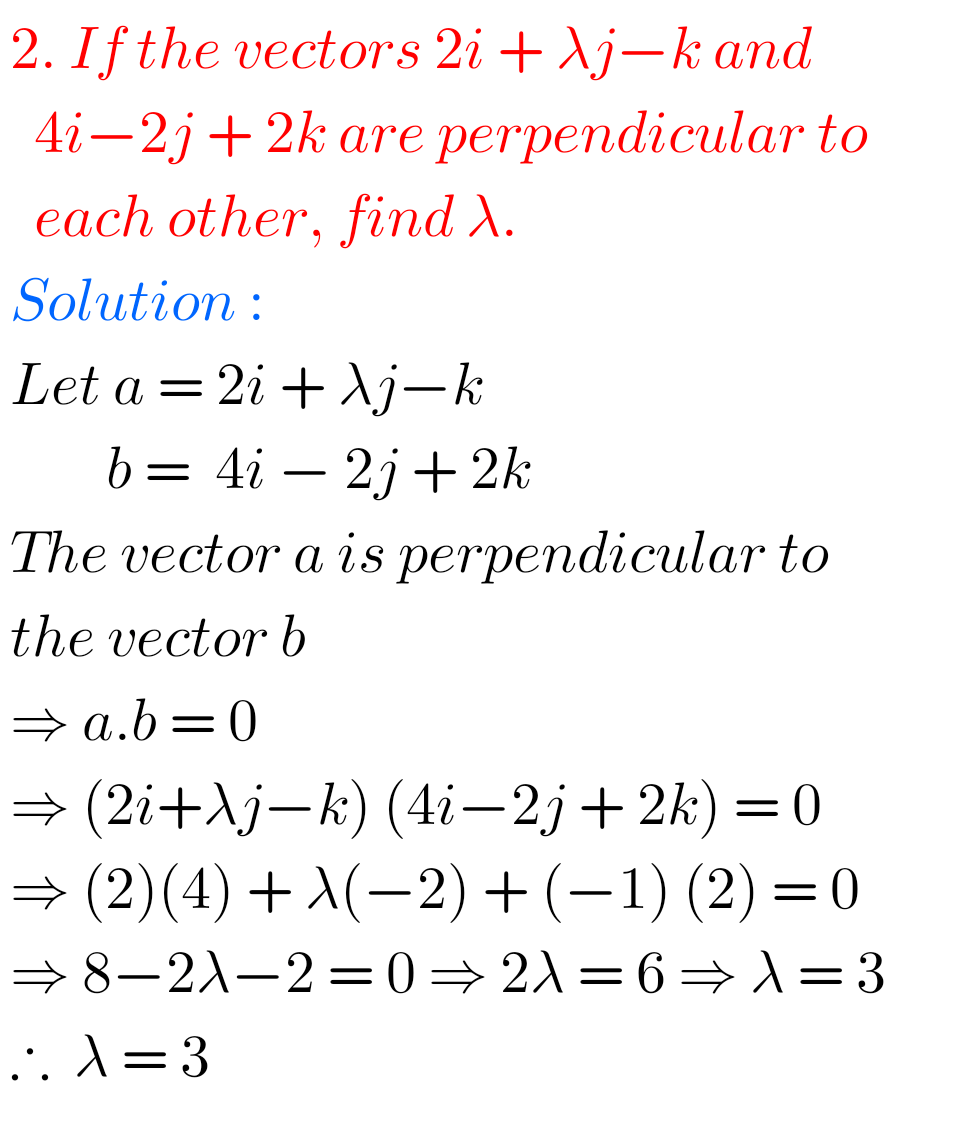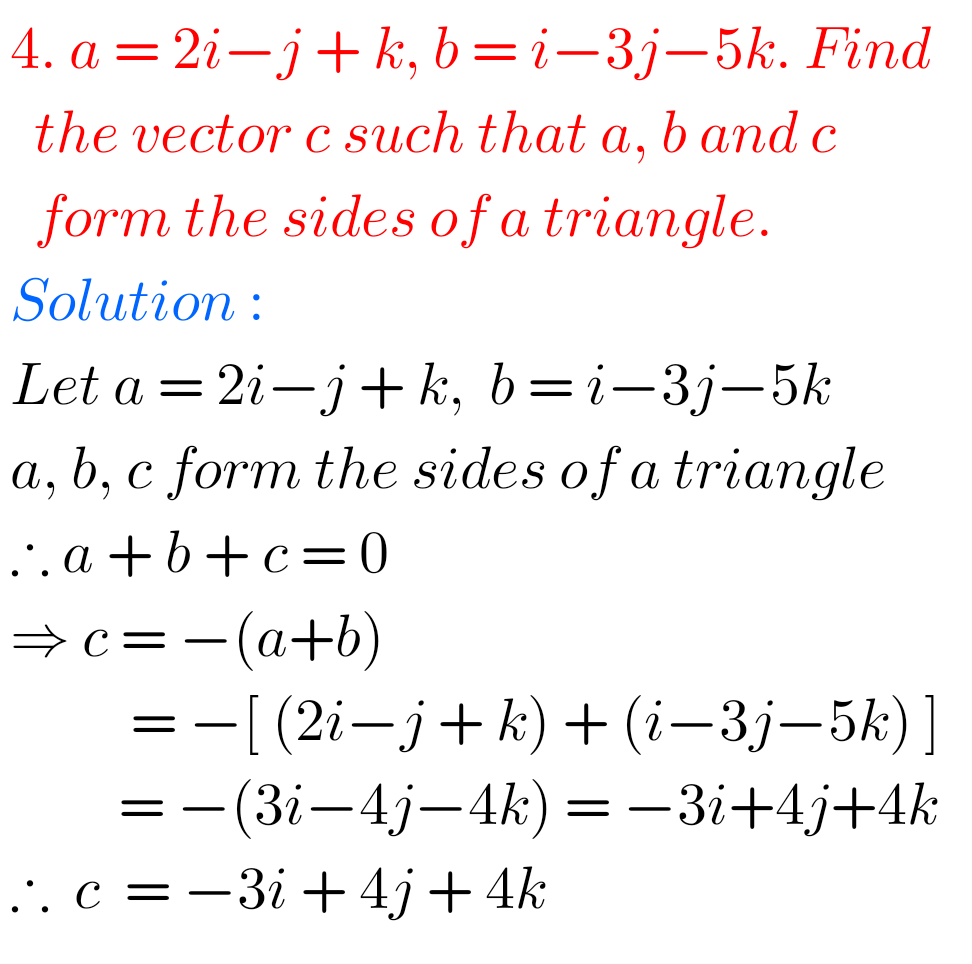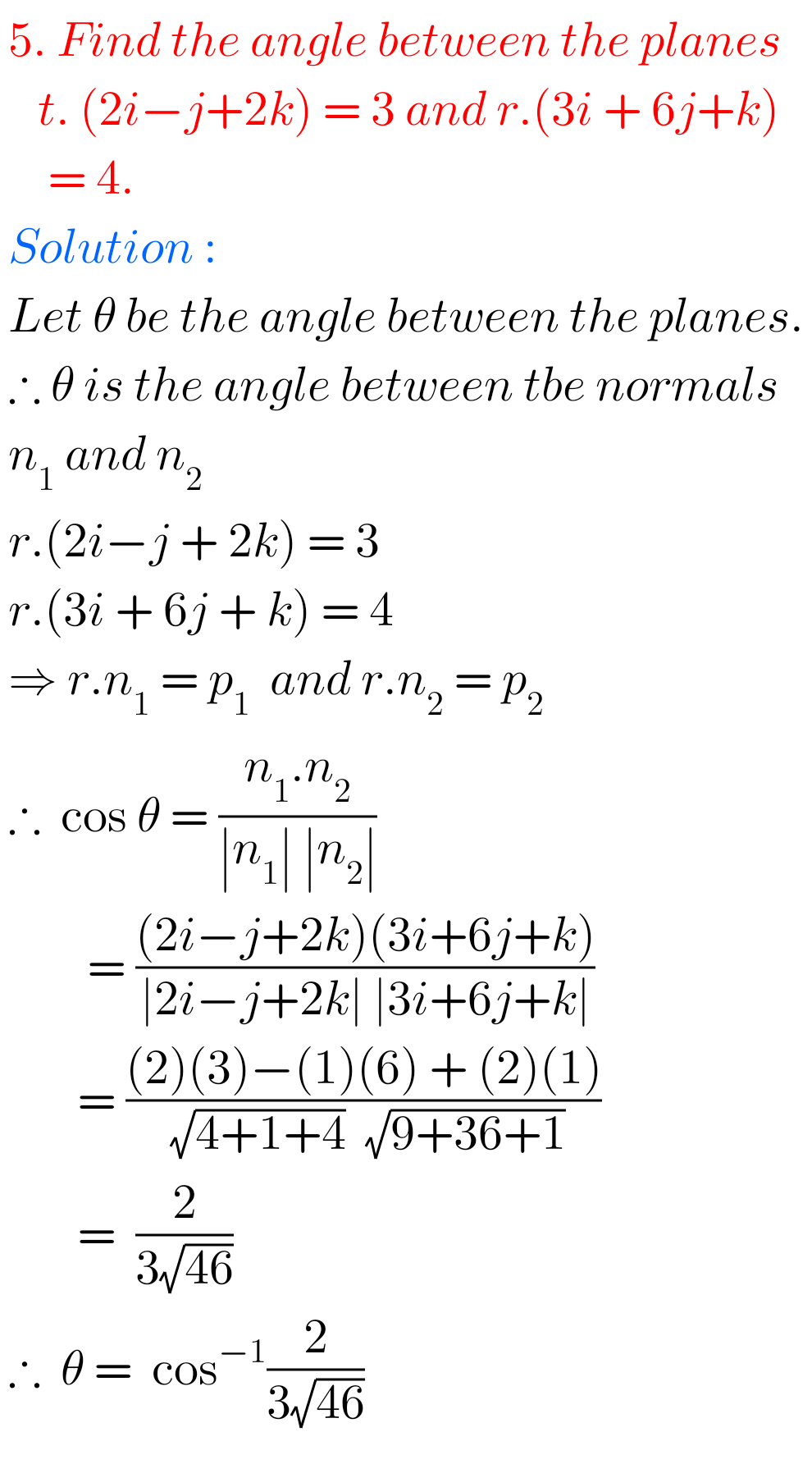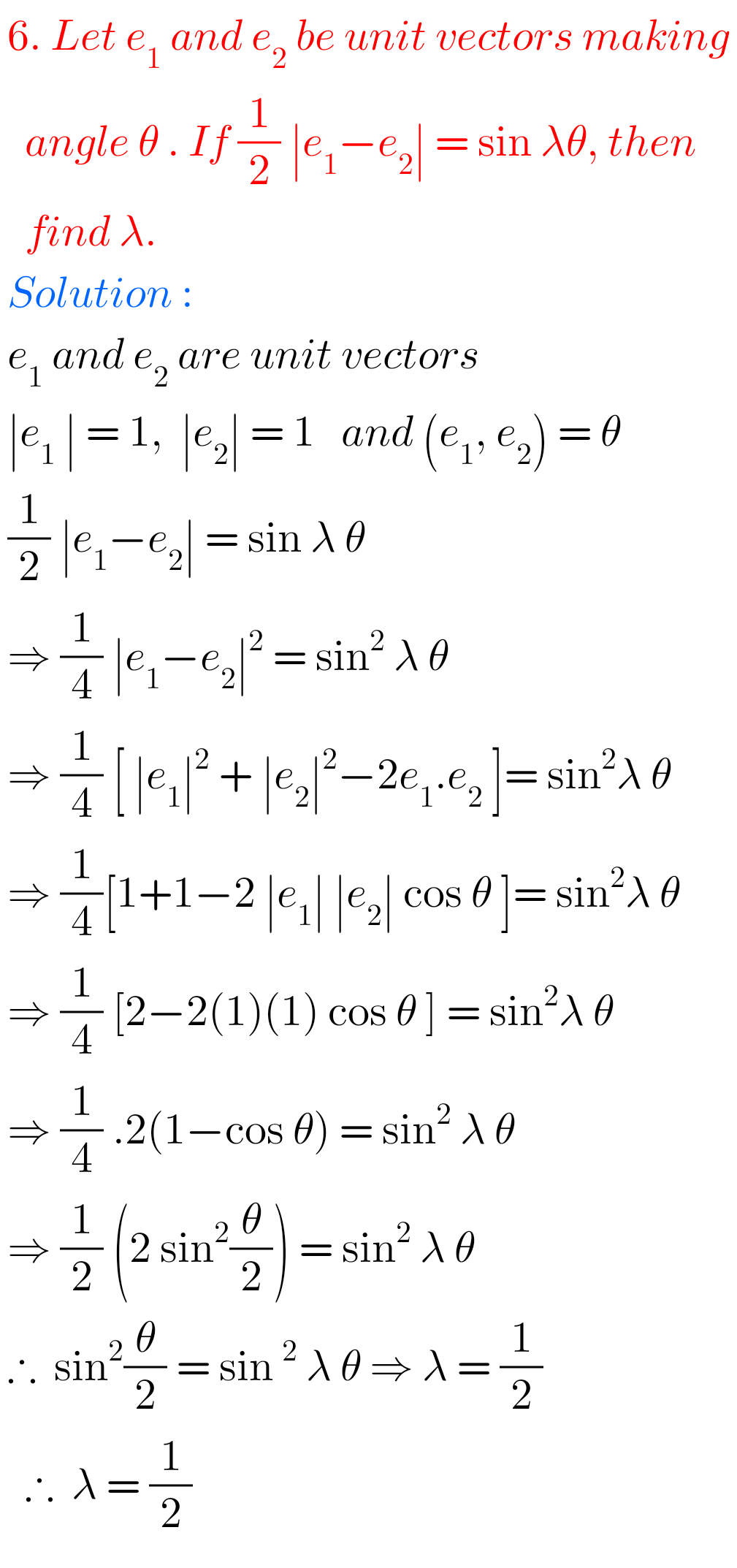#### Maths 1A Solutions for  Exercise 5(a)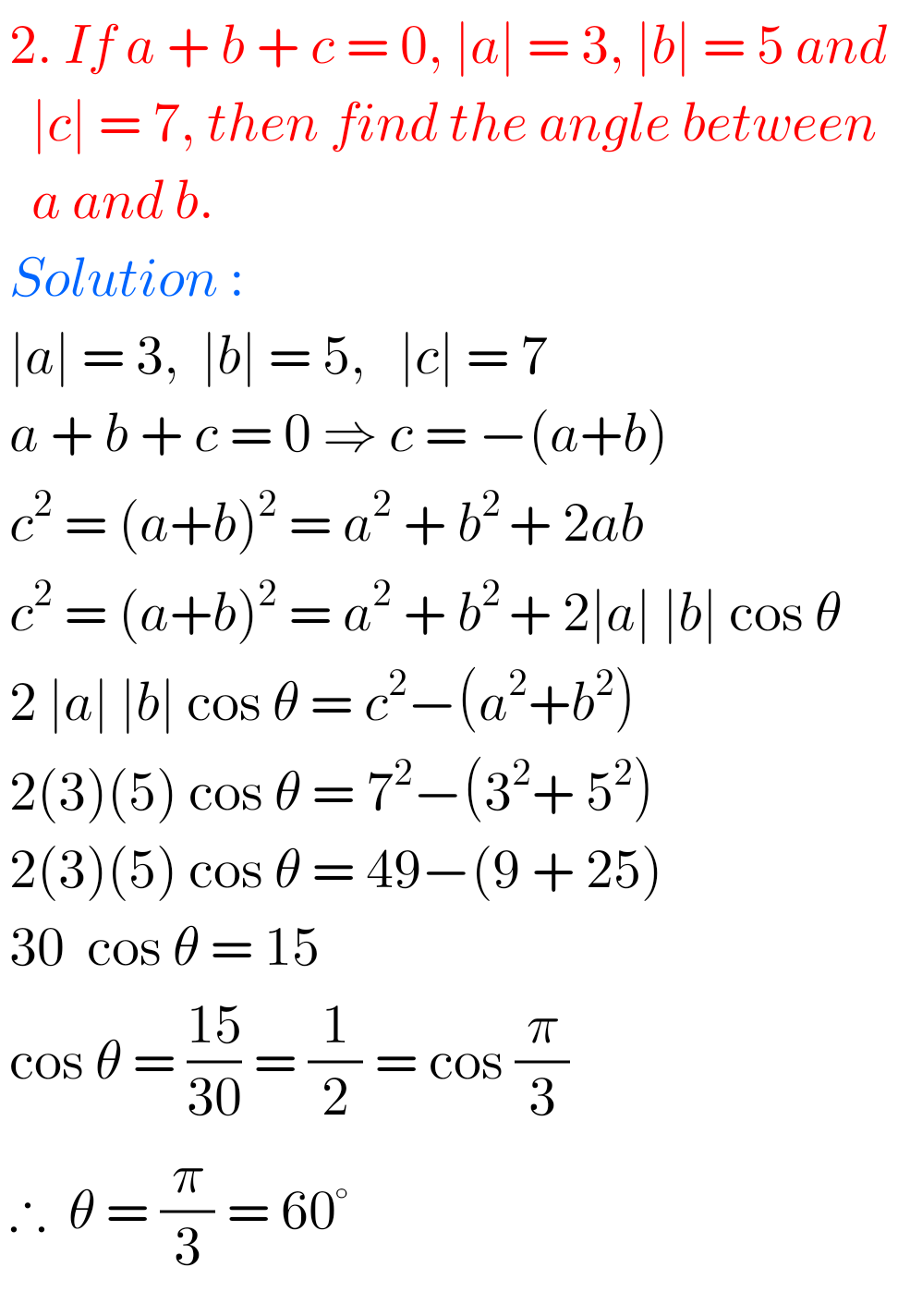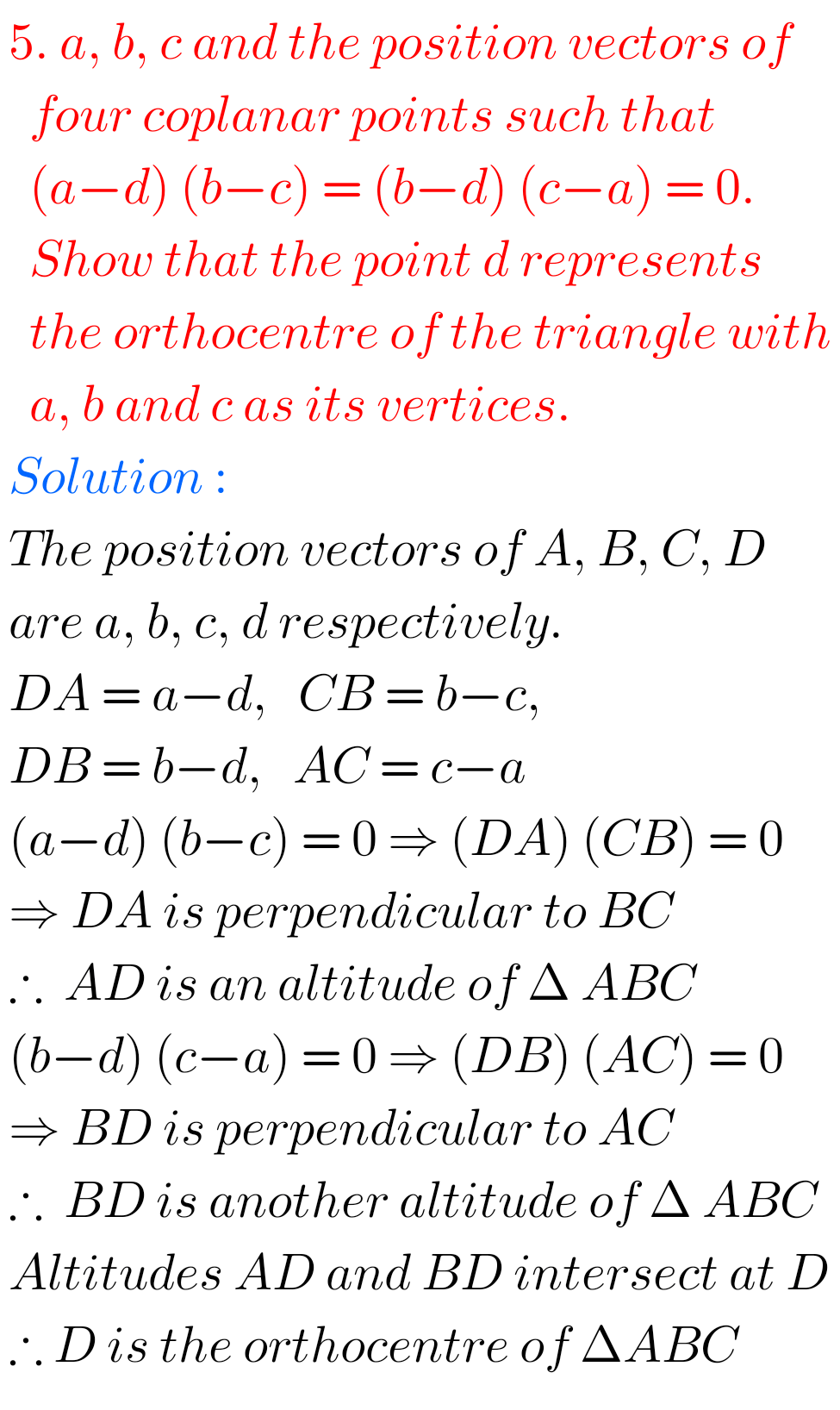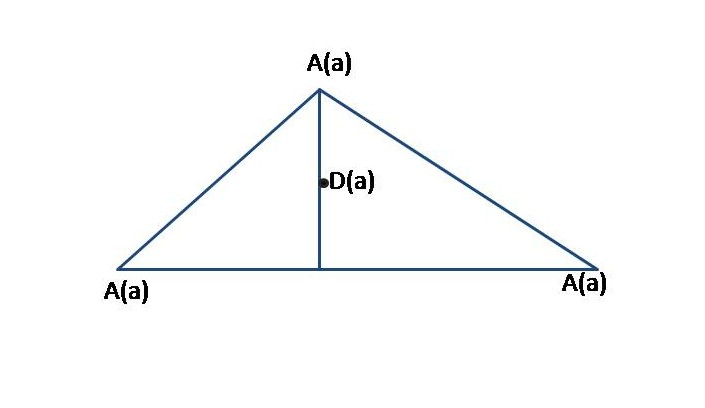## Product of Vectors Exercise 5(a) Solutions Inter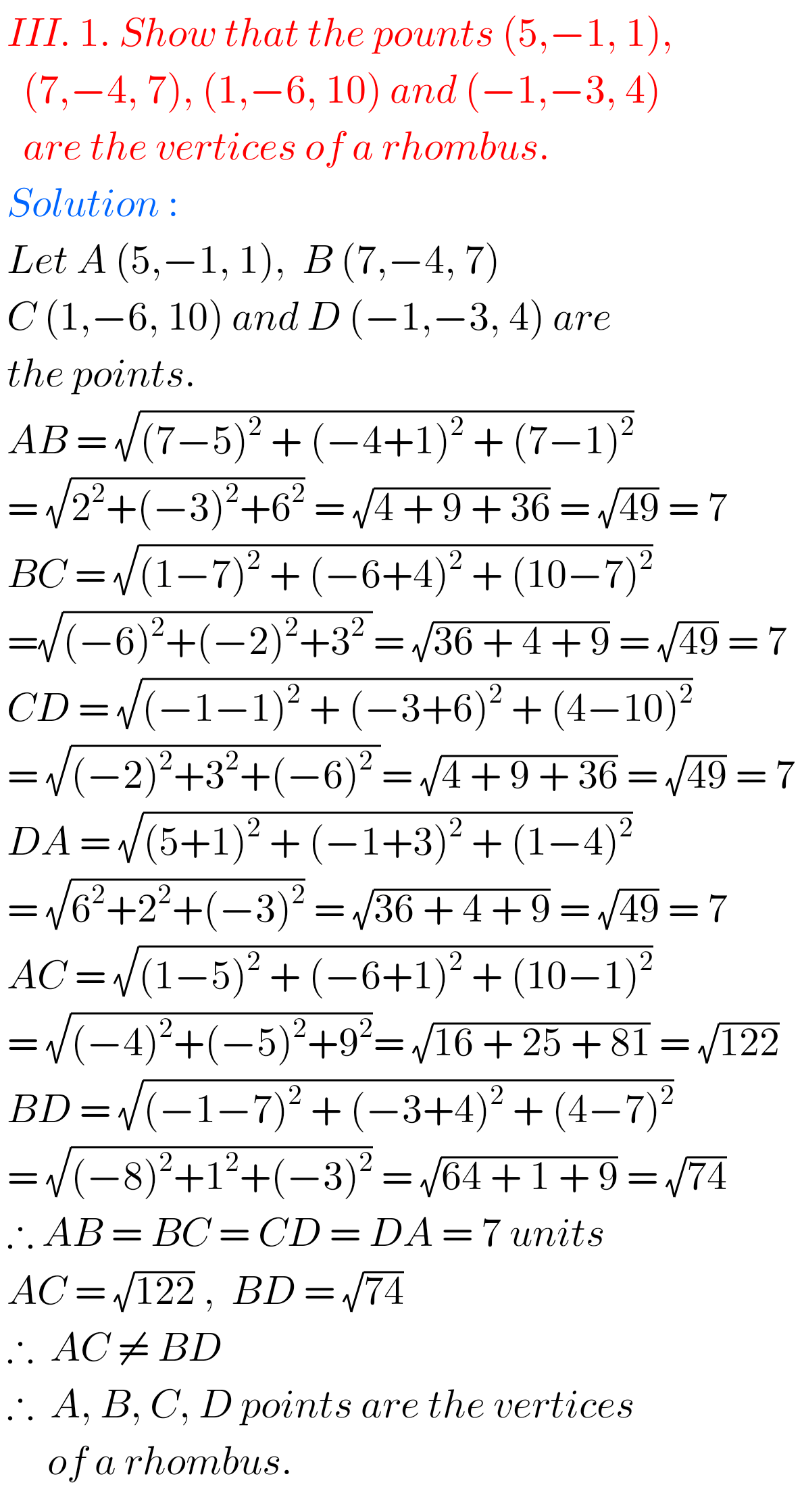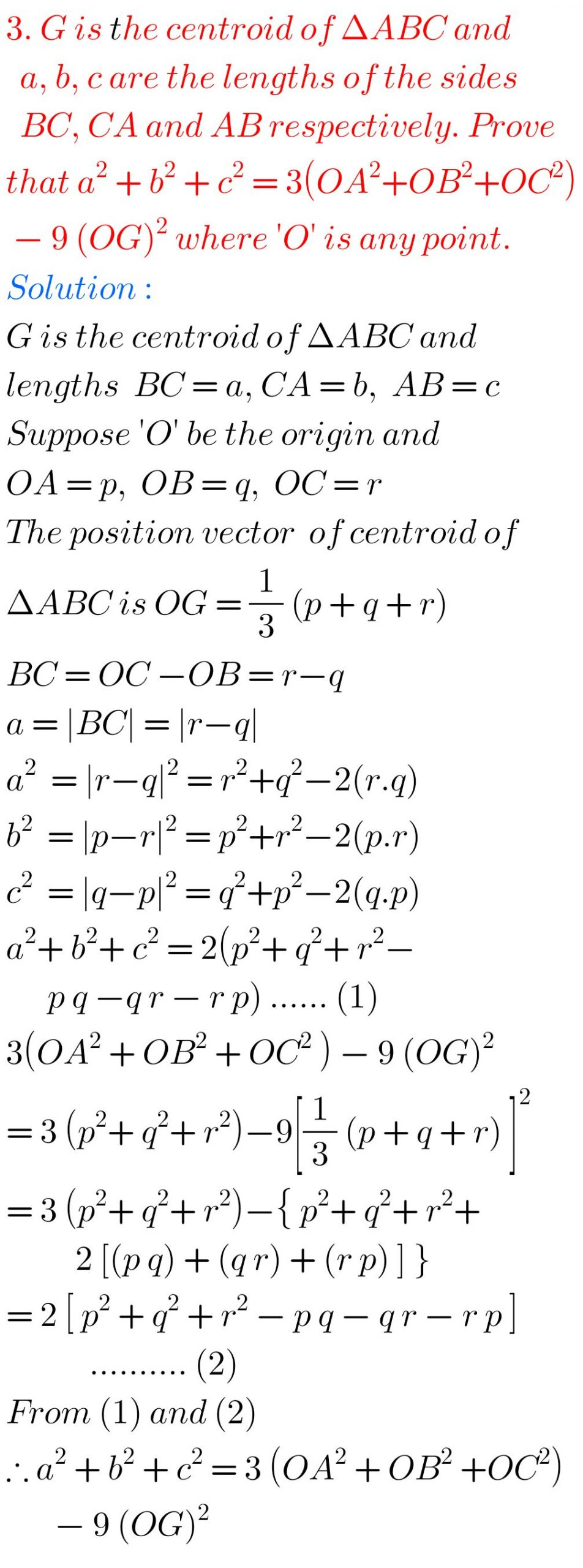Note : Observe the solutions and try them in your own method.

Some more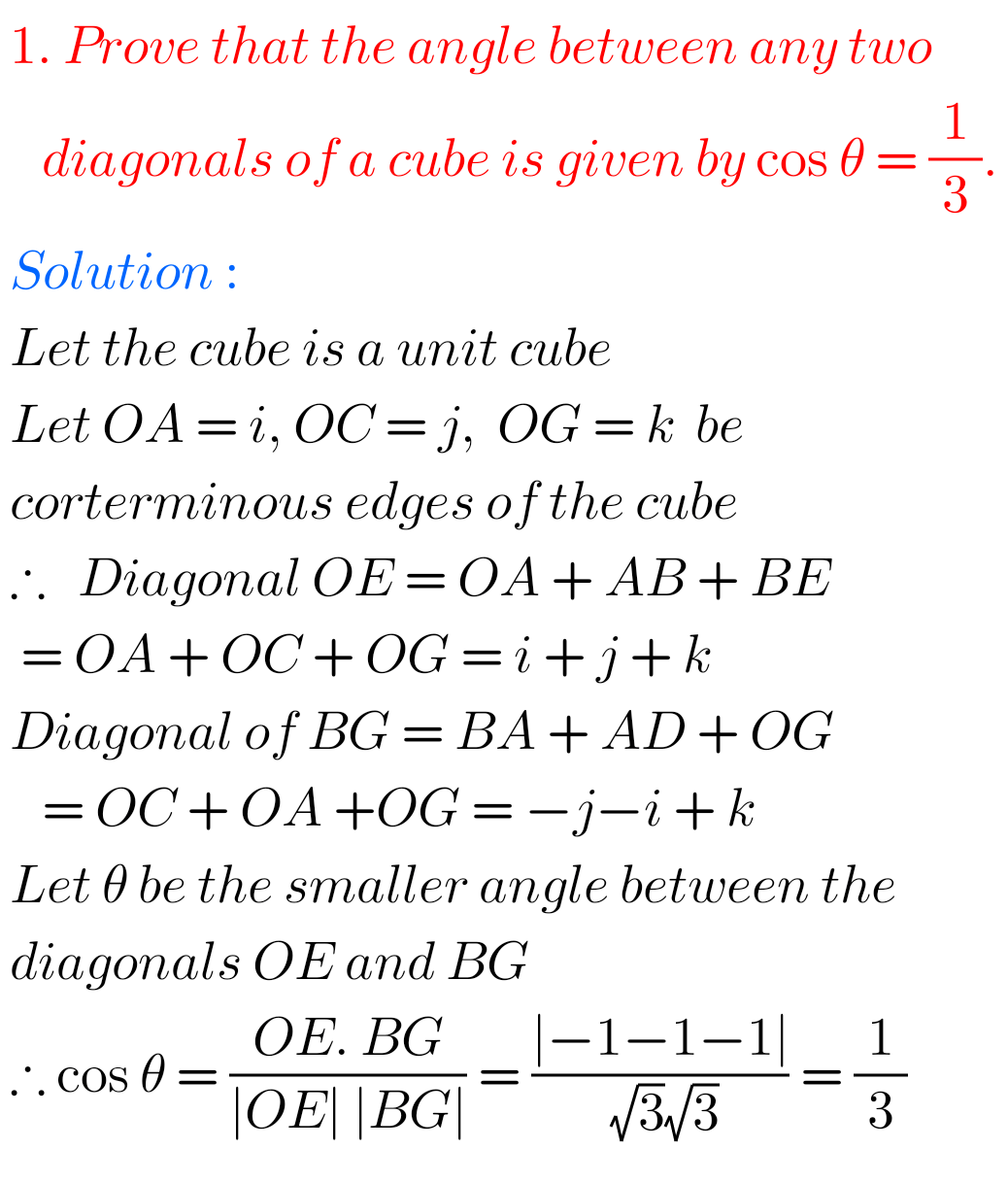You can see the solutions for junior inter maths 1A for examination purpose

2. Matrices